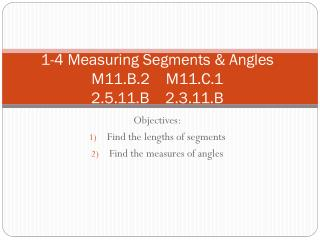DownloadDownload Presentation1-4 Measuring Segments & Angles M11.B.2 M11.C.1 2.5.11.B 2.3.11.B

# 1-4 Measuring Segments & Angles M11.B.2 M11.C.1 2.5.11.B 2.3.11.B

Télécharger la présentation## 1-4 Measuring Segments & Angles M11.B.2 M11.C.1 2.5.11.B 2.3.11.B

- - - - - - - - - - - - - - - - - - - - - - - - - - - E N D - - - - - - - - - - - - - - - - - - - - - - - - - - -
##### Presentation Transcript

1. 1-4 Measuring Segments & AnglesM11.B.2 M11.C.12.5.11.B 2.3.11.B Objectives: Find the lengths of segments Find the measures of angles

2. Here is a Ruler… • What is the distance between points C and D? | | | | | | | | | | 1 2 3 4 5 6 7 8 9 10 C D

3. Postulate 1-5: Ruler Postulate • The points of a line can be put into one-to-one correspondence with the real numbers so that the distance between any two points is the absolute value of the difference of the corresponding numbers. A B . . The length of AB = |a-b| **Think back to our ruler…|2-5| = 3 and |5-2| = 3

4. Vocabulary • Congruent Segments - Two segments with the same length. **congruent symbol (=) Ex) If AB = CD, then AB = CD ~ ~ B A 2 cm B A C 2 cm D C D

5. Example 1: Comparing Segment Lengths • Find which two of the segments XY, ZY and ZW are congruent. X Y Z W 6

6. Postulate 1-6 : Segment Addition Postulate • If three points A, B, and C are collinear and B is between A and C, then AB + BC = AC . A B C

7. Example 2: Segment Addition Postulate • If XY = 10, YZ = 6 and ZW = 8, what is XW? X Y Z W If MP = 37 and NP = 25, what is MN? M N P

8. Example 3: Using the Segment Addition Postulate If AB = 25, find the value of x. Then find AN and NB. . 2x - 6 x + 7 A N B

9. Vocabulary • Midpoint – a point that divides a segment into two congruent segments. A midpoint “bisects” the segment. A B C .

10. Example 4: Using Midpoint • M is the midpoint of NO and NM = 12. Find MO and NO. DRAW A PICTURE!!

11. Example 5: Finding Lengths • M is the midpoint of RT. Find RM, MT and RT. . 5x + 9 8x - 36 R M T

12. Vocabulary • Angle (/)- formed by two rays with the same endpoint. The rays are the sides of the angle. Endpoint is the vertex. • Label angles by their sides or vertex. . T . B Q

13. Example 6: Naming Angles • Name in 4 Ways . T 3 . B Q

14. Classifying Angles • Acute Angle 0<x<90 • Right Angle x= 90 • Obtuse Angle 90<x<180 • Straight Angle x = 180 **To indicate the size or degree measure of an angle, write a lowercase “m” in front of the angle symbol. Example:

15. Example 7: Measuring & Classifying Angles • Classify the Angles 1 2

16. Vocabulary • Congruent Angles – Angles with the same measure. Ex) m<1 = m<2, then <1 = <2 ~

17. Postulate 1-8: Angle Addition Postulate • If point B is in the interior of <AOC, then m<AOB + m<BOC = m<AOC • If <AOC is a straight angle, then m<AOB + m<BOC = 180 A B O C B A C O

18. Example 8: Using the Angle Addition Postulate • Suppose that m<1 = 42 and the m<ABC = 88. Find m<2. A 1 2 B C

19. Example 9: Angle Addition Postulate • If m < DEG = 145, find m < GEF. G D E F